• Pytorch-均方差损失函数和交叉熵损失函数
千次阅读
2020-10-10 17:19:00

### 1.均方差损失函数mse_loss()

均方差损失函数是预测数据和原始数据对应点误差的平方和的均值。

$MSE=\frac{1}{N}( y^−y)^2$

N为样本个数，y'为预测数值，y为正确数值。

代码实例：

import torch
import torch.nn.functional as F

if __name__ == '__main__':
data=torch.tensor([1.0,3.0])
loss=F.mse_loss(torch.tensor([1.0,1.0]),data)
print(loss)
# [(1-1)^2+(3-1)^2]/2  = 2

data1=torch.tensor([2.0,3.0])
loss=F.mse_loss(torch.tensor([1.0,1.0]),data1)
print(loss)
# [(2-1)^2+(3-1)^2]/2  = 2.5

输出结果

tensor(2.)
tensor(2.5000)

### 2.交叉熵损失函数cross_entropy()：相比mse_loss()梯度更大了，优化更快了

先引入熵的概念，熵是衡量分布是否稳定的一个概念，衡量一个分布的信息熵的计算公式如下：log默认以2为底

$Entropy(p)=-\sum_{i=1}^{n} p(i)log p(i)$

衡量一个分布的信息熵的实例化代码如下：

import torch

if __name__ == '__main__':
# 交叉熵一般用于分类问题，如果下面四个数据代表四个类别的比例，
# 四个类别的比例都相同，这里的熵很高，就不容易判断。
data=torch.tensor([0.25,0.25,0.25,0.25])
# 输出熵
print('data的熵为',-(data*torch.log2(data)).sum())
# 熵越高，越不容易确定

# 第四个类别的比例为0.97，这里的熵也很低，就比较容易确定。
data1=torch.tensor([0.01,0.01,0.01,0.97])
# 输出熵
print('data1的熵为',-(data1*torch.log2(data1)).sum())
# 熵越低，越容易确定

输出结果

data的熵为 tensor(2.)
data1的熵为 tensor(0.2419)

衡量两个分布的交叉熵的计算公式如下：

$Entropy(p,q)=-\sum_{i=1}^{n} p(i)log q(i)=Entropy(p)+D_{kl}(p|q)$

交叉熵(p,q)=信息熵(p)+相对熵(p|q),相对熵又称为kl散度,散度越小，p分布和q分布就越接近 p(i)代表的是正确值 q(i)代表的是预测值

交叉熵损失函数经常出现在分类问题中，因为分类问题需要计算各类别的概率，所以交叉熵损失函数经常与sigmoid()和softmax()激活函数搭配使用。python 机器学习 深度学习 人工智能
更多相关内容
• 均方差损失函数和交叉熵损失函数是比较常用的损失函数 分类中常用交叉熵？ MSE 均方误差损失也是一种比较常见的损失函数，其定义为： Cross Entropy Loss Function 二分类 在二分的情况下，模型最后需要预测的结果...

均方差损失函数和交叉熵损失函数是比较常用的损失函数

分类中常用交叉熵？

# MSE

均方误差损失也是一种比较常见的损失函数，其定义为：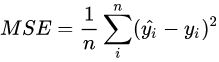# Cross Entropy Loss Function

### 二分类

在二分的情况下，模型最后需要预测的结果只有两种情况，对于每个类别我们的预测得到的概率为P和 1-P ，此时表达式为：其中：
yi—— 表示样本i的label，正类为 1，负类为0
pi—— 表示样本i预测为正类的概率

### 多分类

多分类的情况实际上就是对二分类的扩展：其中：
M——类别的数量
yic——符号函数(0或者1），如果样本 i的真实类别等于C取1 ，否则取0
pic——观测样本 i属于类别C的预测概率

# 计算流程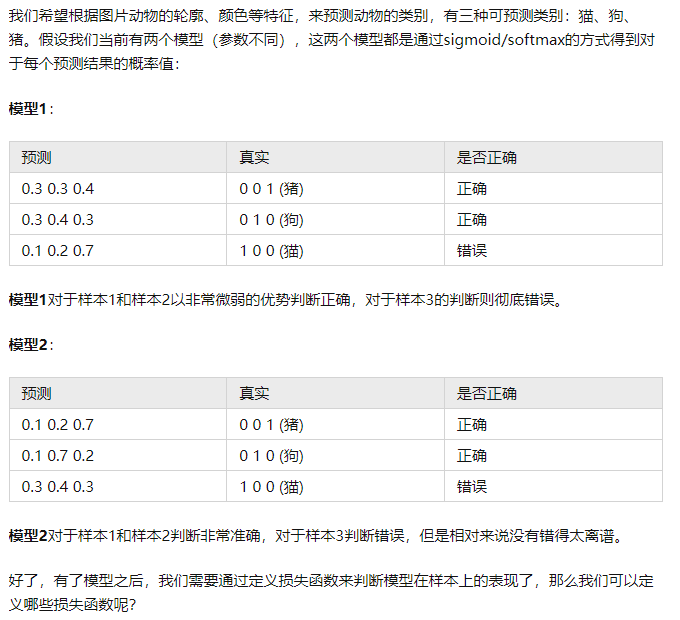### MSE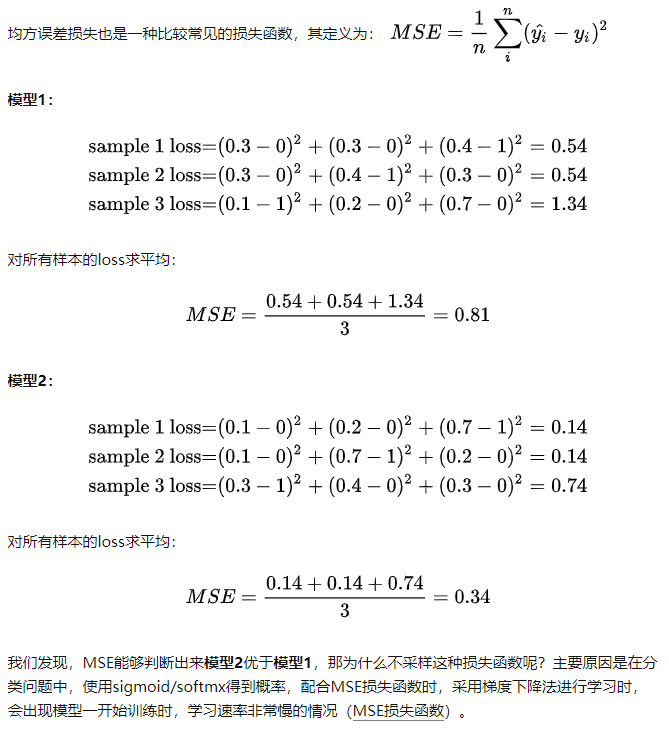### 交叉熵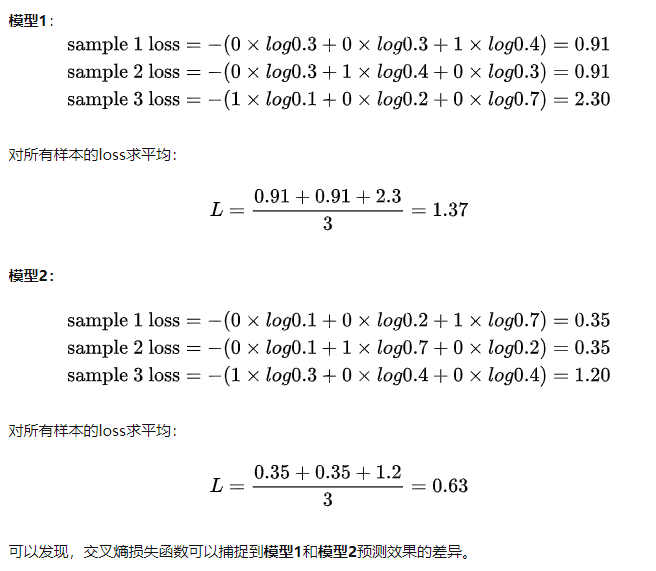# 使用交叉熵的原因

### 交叉熵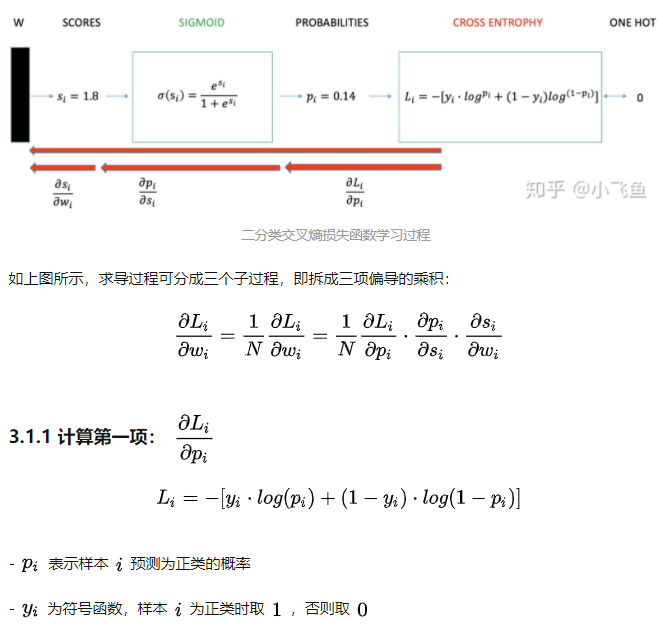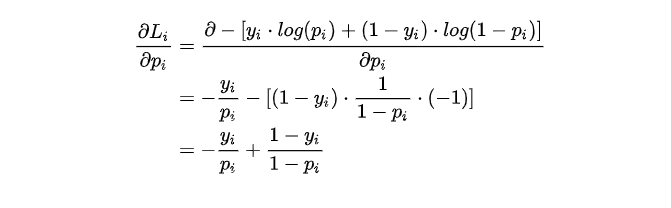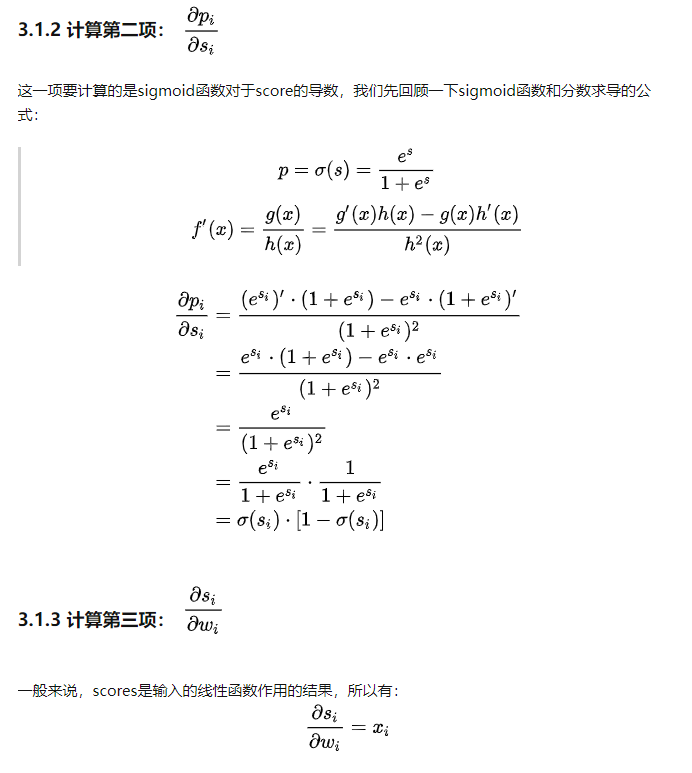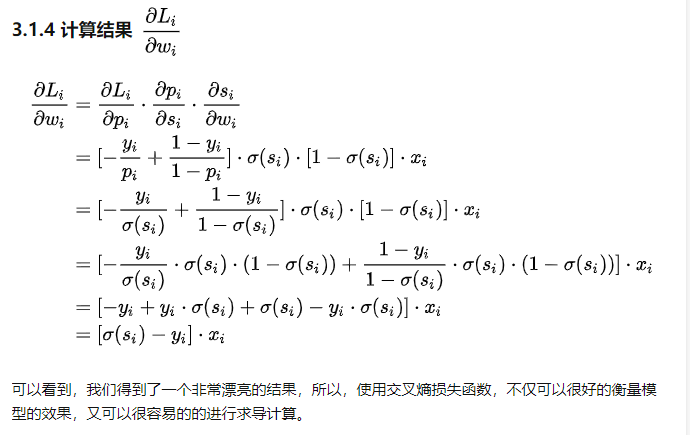### 结论：

在用梯度下降法做参数更新的时候，模型学习的速度取决于两个值：一、学习率；二、偏导值。其中，学习率是我们需要设置的超参数，所以我们重点关注偏导值。从上面的式子中，我们发现，偏导值的大小取决于x 和 sigmod值与y的差 ，我们重点关注后者，后者的大小值反映了我们模型的错误程度，该值越大，说明模型效果越差，但是该值越大同时也会使得偏导值越大，从而模型学习速度更快。所以，使用逻辑函数得到概率，并结合交叉熵当损失函数时，在模型效果差的时候学习速度比较快，在模型效果好的时候学习速度变慢。

### MSE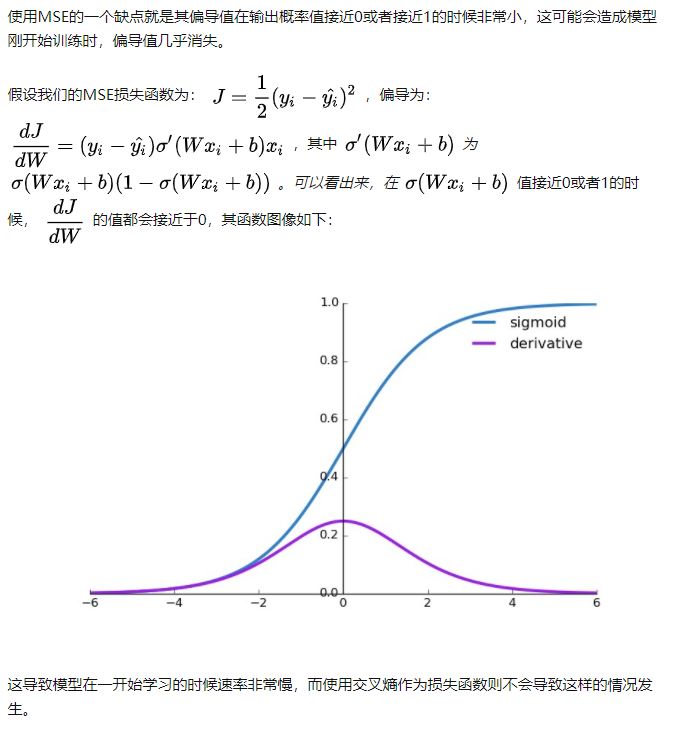参考飞鱼

# 交叉损失函数的由来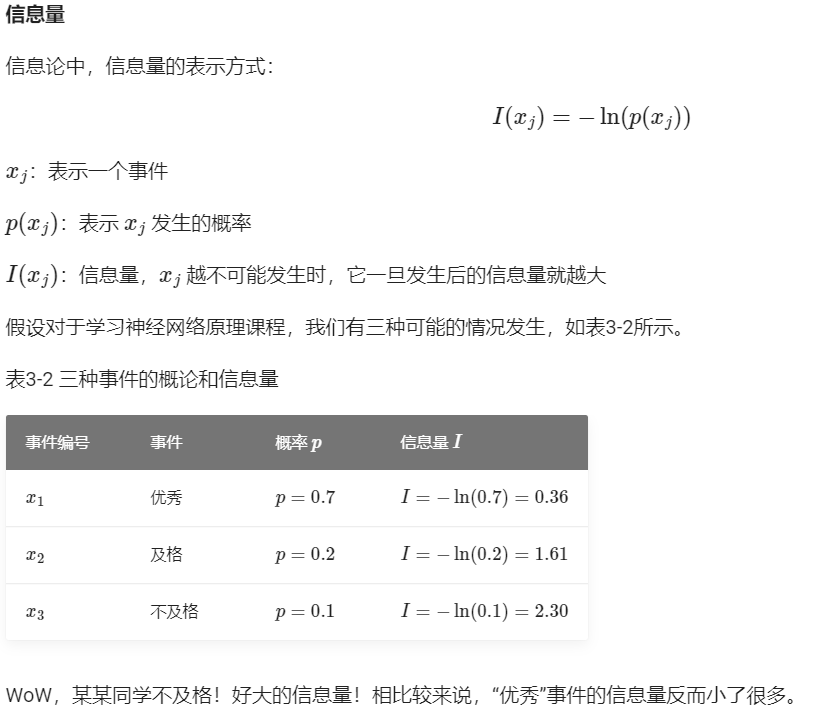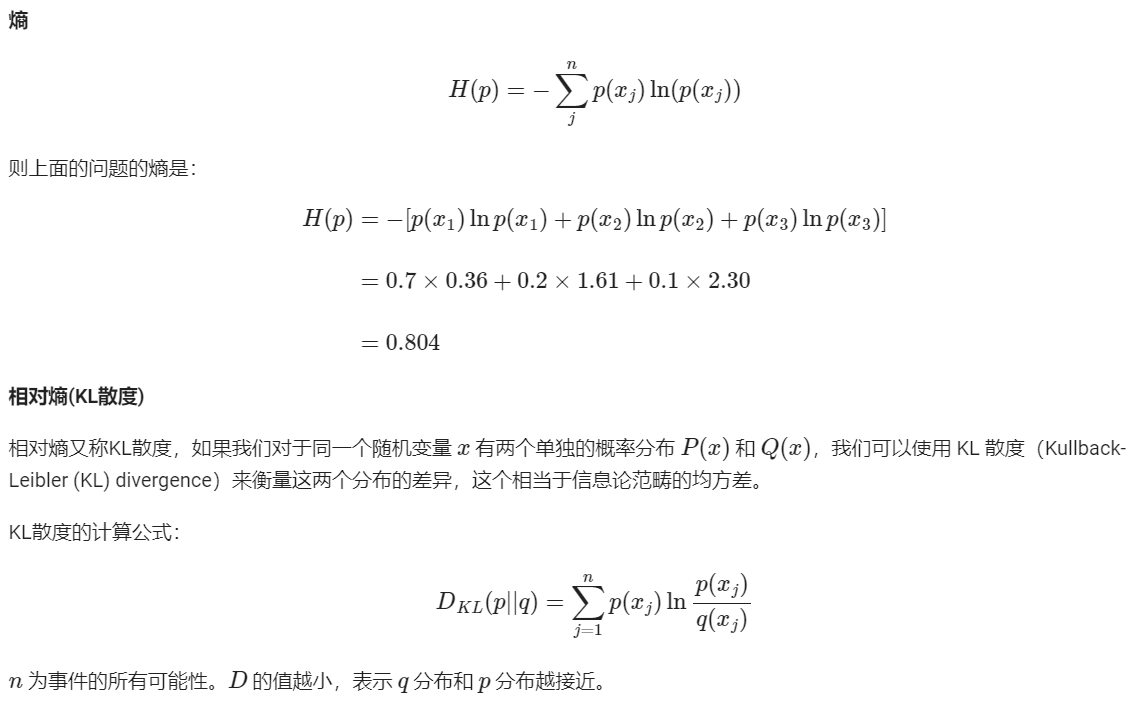利用相对熵来比较两个分布的差异性，所以可以用来比较预测和真实值的差异性，上公式中的P代表着真实值，q代表着预测值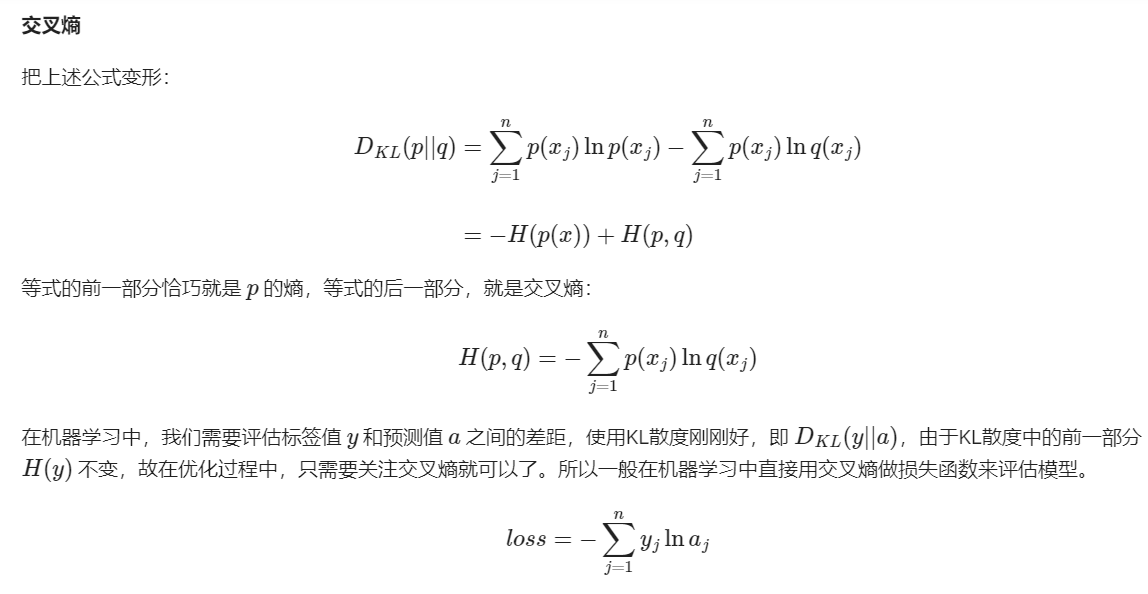其中P的熵是一个定值 要想预测与真实差异小 相对熵需要越小 所以交叉熵需要越小 推出利用交叉熵来代表模型的损失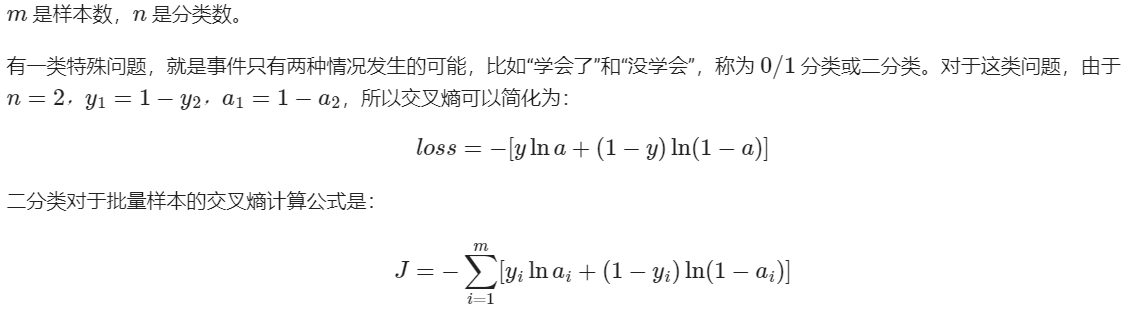展开全文深度学习 机器学习
• 1.均方差损失函数(Mean Squared Error) 均方差损失函数是预测数据和原始数据对应点误差的平方和的均值。计算方式也比较简单 MSE=1N(y^−y)2MSE = \frac{1}{N}(\hat y - y) ^ 2MSE=N1​(y^​−y)2 其中，N为样本个数...

项目github地址：bitcarmanlee easy-algorithm-interview-and-practice
欢迎大家star，留言，一起学习进步

## 1.均方差损失函数(Mean Squared Error)

均方差损失函数是预测数据和原始数据对应点误差的平方和的均值。计算方式也比较简单
M S E = 1 N ( y ^ − y ) 2 MSE = \frac{1}{N}(\hat y - y) ^ 2

其中，N为样本个数。

## 2.交叉熵损失函数(Cross Entropy Error Function)

在分类问题中，尤其是在神经网络中，交叉熵函数非常常见。因为经常涉及到分类问题，需要计算各类别的概率，所以交叉熵损失函数又都是与sigmoid函数或者softmax函数成对出现。

比如用神经网络最后一层作为概率输出，一般最后一层神经网络的计算方式如下：
1.网络的最后一层得到每个类别的scores。
2.score与sigmoid函数或者softmax函数进行计算得到概率输出。
3.第二步得到的类别概率与真实类别的one-hot形式进行交叉熵计算。

二分类的交叉熵损失函数形式
∑ − y i l o g ( y ^ i ) − ( 1 − y i ) l o g ( 1 − y ^ i ) \sum -y_ilog(\hat y_i) - (1-y_i)log(1-\hat y_i)
上面的 y i y_i 表示类别为1, y ^ i \hat y_i 表示预测类别为1的概率。

而多分类的交叉熵损失函数形式为
− ∑ i = 1 n y i l o g ( y ^ i ) -\sum_{i=1} ^n y_i log(\hat y_i)

上面的式子表示类别有n个。单分类问题的时候，n个类别是one-hot的形式，只有一个类别 y i = 1 y_i=1 ，其他n-1个类别为0。

## 3.MSE与sigmoid函数不适合配合使用

MSE的loss为
M S E = − 1 N ( y ^ − y ) 2 MSE =- \frac{1}{N}(\hat y - y) ^ 2
如果其与sigmoid函数配合使用，偏导数为
∂ L o s s i ∂ w = ( y − y ^ ) σ ′ ( w x i + b ) x i \frac{\partial Loss_i}{\partial w} = (y - \hat y) \sigma '(wx _i+ b)x_i
其中
σ ′ ( w x i + b ) = σ ( w x i + b ) ( 1 − σ ( w x i + b ) ) \sigma '(wx _i+ b) = \sigma (wx _i+ b) (1 - \sigma (wx _i+ b))

于是，在 σ ( w x i + b ) \sigma (wx _i+ b) 的值接近0或者1的时候，其导数都接近0。这样会导致模型一开始的学习速度非常慢，所以MSE一般不会与sigmoid函数配合使用。

## 4.交叉熵损失函数与sigmoid函数配合使用

交叉熵损失函数与sigmoid函数配合使用，最终损失函数求导的结果为
∂ L o s s i ∂ w = ( y ^ i − y i ) x i \frac{\partial Loss_i}{\partial w} = (\hat y_i - y_i)x_i

由此可见，求导的结果与 y ^ i − y i \hat y_i - y_i x i x_i 的值有关，不会出现模型开始训练速度很慢的现象。

具体的推导过程见参考文献1
交叉熵损失函数求导

## 5.交叉熵损失函数与softmax函数配合使用

前面提到，在神经网络中，交叉熵损失函数经常与softmax配合使用。
L o s s = − ∑ i t i l n y i Loss = - \sum_i t_i lny_i
softmax函数
y i = e i ∑ j e j = 1 − ∑ j ≠ i e j ∑ j e j y_i = \frac{e^i}{\sum_j e^j} = 1 - \frac{\sum_{j \neq i} e^j}{\sum_j e^j}

接下来求导

∂ L o s s i ∂ i = − ∂ l n y i ∂ i = − ∑ j e j e i ⋅ ∂ ( e i ∑ j e j ) ∂ i = − ∑ j e j e i ⋅ ( − ∑ j ≠ i e j ) ⋅ ∂ ( 1 ∑ j e j ) ∂ i = ∑ j e j ⋅ ∑ j ≠ i e j e i ⋅ − e i ( ∑ j e j ) 2 = − ∑ j ≠ i e j ∑ j e j = − ( 1 − e i ∑ j e j ) = y i − 1 {\begin{aligned} \frac{\partial Loss_i}{\partial _i} & = - \frac{\partial ln y_i}{\partial _i} \\ & = -\frac{\sum_j e^j}{e^i} \cdot \frac {\partial (\frac{e_i}{\sum_j e^j})}{\partial_i} \\ & = -\frac{\sum_j e^j}{e^i} \cdot (- \sum _ {j \neq i}e^j ) \cdot \frac{\partial( \frac {1} {\sum_j e^j} ) } { \partial _i} \\ & = \frac { \sum_j e^j \cdot \sum_{j \neq i} e^j} {e^i } \cdot \frac { - e^i} { (\sum_j e^j) ^ 2} \\ & = -\frac { \sum_{j \neq i} e^j } { \sum_j e^j } \\ & = -(1 - \frac{ e ^ i } { \sum_j e^j } ) \\ & = y_i - 1 \end{aligned}}

由此可见，交叉熵函数与softmax函数配合，损失函数求导非常简单！

## 参考文献

1.https://blog.csdn.net/bitcarmanlee/article/details/51473567

展开全文sigmoid函数 softmax函数
• 系列博客，原文在笔者所维护的github上：...该函数就是最直观的一个损失函数了，计算预测值和真实值之间的欧式距离。预测值和真实值越接近，两者的均方差就越小。 均方差函数常用于线性回归(linear regression)...

系列博客，原文在笔者所维护的github上：https://aka.ms/beginnerAI
点击star加星不要吝啬，星越多笔者越努力。

## 3.1 均方差函数

MSE - Mean Square Error。

该函数就是最直观的一个损失函数了，计算预测值和真实值之间的欧式距离。预测值和真实值越接近，两者的均方差就越小。

均方差函数常用于线性回归(linear regression)，即函数拟合(function fitting)。公式如下：

$loss = {1 \over 2}(z-y)^2 \tag{单样本}$

$J=\frac{1}{2m} \sum_{i=1}^m (z_i-y_i)^2 \tag{多样本}$

### 3.1.1 工作原理

要想得到预测值a与真实值y的差距，最朴素的想法就是用$$Error=a_i-y_i$$

对于单个样本来说，这样做没问题，但是多个样本累计时，$$a_i-y_i$$有可能有正有负，误差求和时就会导致相互抵消，从而失去价值。所以有了绝对值差的想法，即$$Error=|a_i-y_i|$$。这看上去很简单，并且也很理想，那为什么还要引入均方差损失函数呢？两种损失函数的比较如表3-1所示。

表3-1 绝对值损失函数与均方差损失函数的比较

样本标签值样本预测值绝对值损失函数均方差损失函数
$$[1,1,1]$$$$[1,2,3]$$$$(1-1)+(2-1)+(3-1)=3$$$$(1-1)^2+(2-1)^2+(3-1)^2=5$$
$$[1,1,1]$$$$[1,3,3]$$$$(1-1)+(3-1)+(3-1)=4$$$$(1-1)^2+(3-1)^2+(3-1)^2=8$$
$$4/3=1.33$$$$8/5=1.6$$

可以看到5比3已经大了很多，8比4大了一倍，而8比5也放大了某个样本的局部损失对全局带来的影响，用术语说，就是“对某些偏离大的样本比较敏感”，从而引起监督训练过程的足够重视，以便回传误差。

### 3.1.2 实际案例

假设有一组数据如图3-3，我们想找到一条拟合的直线。

图3-3 平面上的样本数据

图3-4中，前三张显示了一个逐渐找到最佳拟合直线的过程。

• 第一张，用均方差函数计算得到Loss=0.53；
• 第二张，直线向上平移一些，误差计算Loss=0.16，比图一的误差小很多；
• 第三张，又向上平移了一些，误差计算Loss=0.048，此后还可以继续尝试平移（改变b值）或者变换角度（改变w值），得到更小的损失函数值；
• 第四张，偏离了最佳位置，误差值Loss=0.18，这种情况，算法会让尝试方向反向向下。

图3-4 损失函数值与直线位置的关系

第三张图损失函数值最小的情况。比较第二张和第四张图，由于均方差的损失函数值都是正值，如何判断是向上移动还是向下移动呢？

在实际的训练过程中，是没有必要计算损失函数值的，因为损失函数值会体现在反向传播的过程中。我们来看看均方差函数的导数：

$\frac{\partial{J}}{\partial{a_i}} = a_i-y_i$

虽然$$(a_i-y_i)^2$$永远是正数，但是$$a_i-y_i$$却可以是正数（直线在点下方时）或者负数（直线在点上方时），这个正数或者负数被反向传播回到前面的计算过程中，就会引导训练过程朝正确的方向尝试。

在上面的例子中，我们有两个变量，一个w，一个b，这两个值的变化都会影响最终的损失函数值的。

我们假设该拟合直线的方程是y=2x+3，当我们固定w=2，把b值从2到4变化时，看看损失函数值的变化如图3-5所示。

图3-5 固定W时，b的变化造成的损失值

我们假设该拟合直线的方程是y=2x+3，当我们固定b=3，把w值从1到3变化时，看看损失函数值的变化如图3-6所示。

图3-6 固定b时，W的变化造成的损失值

### 3.1.3 损失函数的可视化

#### 损失函数值的3D示意图

横坐标为W，纵坐标为b，针对每一个w和一个b的组合计算出一个损失函数值，用三维图的高度来表示这个损失函数值。下图中的底部并非一个平面，而是一个有些下凹的曲面，只不过曲率较小，如图3-7。

图3-7 W和b同时变化时的损失值形成的曲面

#### 损失函数值的2D示意图

在平面地图中，我们经常会看到用等高线的方式来表示海拔高度值，下图就是上图在平面上的投影，即损失函数值的等高线图，如图3-8所示。

图3-8 损失函数的等高线图

如果还不能理解的话，我们用最笨的方法来画一张图，代码如下：

    s = 200
W = np.linspace(w-2,w+2,s)
B = np.linspace(b-2,b+2,s)
LOSS = np.zeros((s,s))
for i in range(len(W)):
for j in range(len(B)):
z = W[i] * x + B[j]
loss = CostFunction(x,y,z,m)
LOSS[i,j] = round(loss, 2)

上述代码针对每个w和b的组合计算出了一个损失值，保留小数点后2位，放在LOSS矩阵中，如下所示：

[[4.69 4.63 4.57 ... 0.72 0.74 0.76]
[4.66 4.6  4.54 ... 0.73 0.75 0.77]
[4.62 4.56 4.5  ... 0.73 0.75 0.77]
...
[0.7  0.68 0.66 ... 4.57 4.63 4.69]
[0.69 0.67 0.65 ... 4.6  4.66 4.72]
[0.68 0.66 0.64 ... 4.63 4.69 4.75]]`

然后遍历矩阵中的损失函数值，在具有相同值的位置上绘制相同颜色的点，比如，把所有值为0.72的点绘制成红色，把所有值为0.75的点绘制成蓝色......，这样就可以得到图3-9。

图3-9 用笨办法绘制等高线图

此图和等高线图的表达方式等价，但由于等高线图比较简明清晰，所以以后我们都使用等高线图来说明问题。

### 代码位置

ch03, Level1

展开全文• 损失函数的一般表示为L(y,f(x))，用以衡量真实值y和预测值f(x)之间不一致的程度，一般越小越好。为了便于不同损失函数的比较，常将其表示为单变量的函数，在回归问题中这个变量为y−f(x)，在分类问题中则为yf(x)。...深度学习
• 在深度学习中，做回归任务时使用的loss多为均方差。公式为 其中：Batch为样本数量，M为网络输出层的元素的个数 实现 loss = tf.nn.l2_loss(x, x') *2.0/ (Batch*M) loss = tf.losses.mean_squared_error(x, ...
• 交叉熵和均方差损失函数的比较交叉熵 熵是香农信息量（）（底数是2）的期望，即为衡量一个样本所需要的平均编码长度，表示为： 其中的pi表示样本的分布，现在如果用一个估计的分布qi来表示求真实分布pi的平均编码...
• 我们定义，将b放到w中，，其中f为激活函数。 总结 对SE而言，要得到一个线性的梯度，必须输出不经过激活函数才行。这样的情况只有线性回归，所以SE较适合做回归问题，而CE更适合做分类问题，在分类问题...
• 均方差损失函数求误差及梯度 完整源码可戳：https://github.com/AKGWSB/Convolution-Neural-Network-Frame-only-based-on-Numpy-/blob/master/Loss.py 损失函数表达式 即 输出减去目标的平方再乘以二分之一 MSE = 1...人工智能 深度学习
• 本文给出均方差损失函数 MSELoss 的定义并求解其在反向传播中的梯度. 相关 系列文章索引 : https://blog.csdn.net/oBrightLamp/article/details/85067981 正文 均方差损失函数 MSELoss 定义简洁, 梯度求导简单, 应用...
• 1. 均方差损失函数(Mean Squared Error, MSE) 均方差损失函数是预测数据和原始数据对应点误差的平方和的均值，公式为： MSE=1N(y^−y)2MSE = \frac{1}{N}(\hat{y}-y)^{2}MSE=N1​(y^​−y)2 N为样本个数。 2. 方差...深度学习 pytorch 机器学习
• 损失函数是网络学习的指挥棒，它引导着网络学习的方向——能让损失函数变小的参数就是好参数。 所以，损失函数的选择和设计要能表达你希望模型具有的性质与倾向。 交叉熵损失函数 交叉熵是用来衡量两个概率分布...神经网络 人工智能 深度学习
• 均方差函数（MSE Mean Square Error） 计算预测值和真实值之间的欧式距离。预测值和真实值越接近，两者的均方差就越小 均方差函数常用于线性回归(linear regression)，即函数拟合(function fitting)。 公式 J(w,b)=...
• 本篇博文主要讲用于分类问题的交叉熵和回归问题的均方差。先来说下分类和回归的区别。机器学习或深度学习领域常见的就是分类和回归，通俗的讲分类就把样品分到事先定义好的n个类别中，解决的是离散量的问题，回归...机器学习
• 损失函数求最小，求方差的最小，和求均方差的最小应该都是一个作用吧。为啥用sum就得不到结果呢，用mean就可以。不理解 import tensorflow.compat.v1 as tf import numpy as np # 使用 NumPy 生成假数据(phony ...tensorflow
• 每幅图上的曲线，显示了随着预测准确率的提高，交叉熵损失和均方差损失的变化情况。 其中横坐标为准确率，范围在0到1之间，纵坐标为损失。 对比的类别数范围在[2, 100]，每个类别使用的样本量是1000组。 可以发现，...
• 然而，将均方差作为损失函数是通过推导得到的。 首先，线性回归属于普通线性模型，而普通线性回归中的误差项满足下面四个假设： 零均值假设：误差项是期望为零的随机变量，即E(e)=0E(e)=0E(e)=0 不变方差假设：误差...
• 激活函数与损失函数关系 激活函数先进行0-1区间，再输入损失函数与label进行误差计算，最后反向传播 常用交叉熵的原因 A)、原因在于交叉熵函数配合输出层的激活函数如sigmoid或...3、交叉熵（分类）、均方差（回python airflow r语言
• ## 常用损失函数小结

万次阅读 多人点赞 2018-05-27 11:01:58
一、摘要本文主要总结一下常见的损失函数，包括：MSE均方误差损失函数、SVM合页损失函数、Cross Entropy交叉熵损失函数、目标检测中常用...二、均方误差损失2.1 均方差损失函数的定义：均方差损失函数常用在最小二乘...
• 在《深度学习（一）：DNN前向传播算法和反向传播算法》中，我们对DNN的前向反向传播算法的使用做了总结。里面使用的损失函数是均方差，而激活函数是sigmoid。...在讲反向传播算法时，我们用均方差损失函数和Sigmo...DNN 激活函数 ReLU SoftMax
• MSE为非凸函数，存在多个极值点，很可能找不到最优解 ，会出现局部最优，不适合作为损失函数 Convex Optimization——凸函数 - 知乎 (zhihu.com)https://zhuanlan.zhihu.com/p/56876303机器学习
• 0-1损失函数（zero-one loss）、绝对值损失、指数损失、Hinge 损失、感知损失、交叉熵损失（CE）、权重交叉熵损失（WCE）、Focal Loss 均方差、平均绝对误差、Huber Loss（Smooth L1 Loss）、分位数回归损失、IoU ...深度学习
• 本文主要总结一下常见的损失函数，包括：MSE均方误差损失函数、Cross Entropy交叉熵损失函数、目标检测中常用的Smooth L1损失函数。其中还会涉及到梯度消失、梯度爆炸等问题：ESM均方误差+Sigmoid激活函数会导致学习...
• ## pytorch损失函数

千次阅读 2019-07-02 18:19:43
学习深度学习的过程中一直会遇到损失函数，均方损失函数、交叉熵损失函数、负对数似然损失函数 有些时候觉得有点不清晰，所以总结、梳理一下，加深自己的理解 MSELoss损失函数 # MSELoss有一个参数 reduction='sum'...pytorch NLLLoss
• 也就是说在模型输出与真实值的误差服从高斯分布的假设下，最小化均方差损失函数与极大似然估计本质上是一致的，因此在这个假设能被满足的场景中（比如回归），方差损失是一个很好的损失函数选择；当这个假设没能被...
• 来源：七月在线实验室本文约4300字，建议阅读9分钟。本文将介绍机器学习、深度学习中分类与回归常用的几种损失函数。机器学习中的监督学习本质上是给定一系列训练样本，尝试学习的映射关系...算法 python 机器学习 人工智能 深度学习
• # mean（（y_prd-y_true）** 2） 均方差损失函数 return tf.reduce_mean(tf.square(y_prd - y_true)) # 模型的编译 设置损失函数 优化器 model.compile(loss=custom_loss, # 使用自定义损失函数 optimizer='sgd......